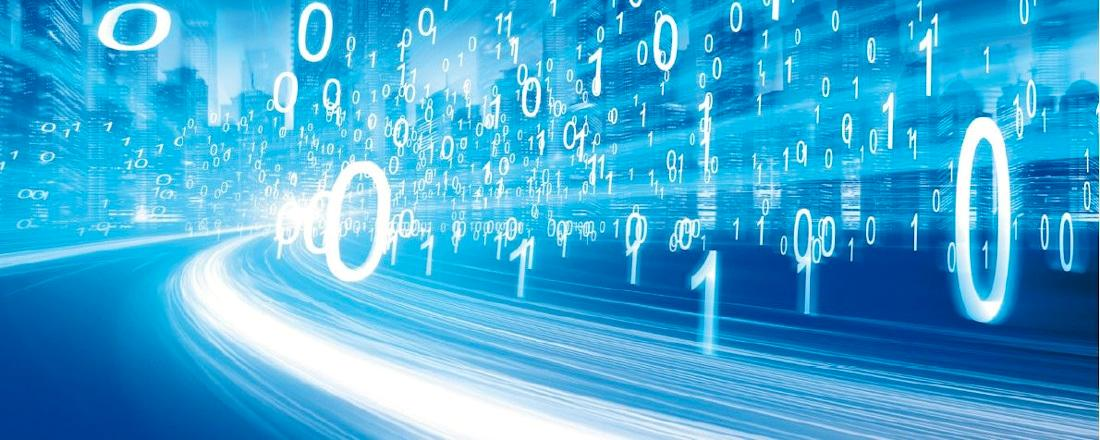See here my previous teaching activities.

# Practical Scientific Computing

This is the website for the course Practical Scientific Computing at Ecole des Ponts. Lecture notes and assignments will be posted here weekly.

## Practical information

Lectures
Friday from 13:30 to 16:15.
Assessment
100% assignments + oral discussion.
Reference textbook

## Lecture notes

• Lecture notes: [pdf]

• Syllabus: [pdf]

• Introduction: [pdf]

• Chapter 1: Floating point arithmetic: [pdf]

• Chapter 2: Interpolation and approximation: [pdf]

• Chapter 3: Numerical integration: [pdf]

• Chapter 4: Solving linear equations: [pdf]

• Chapter 5: Solving nonlinear equations: [pdf]

• Chapter 6: Calculating eigenvalues and eigenvectors: [pdf]

• Chapter 7: Numerical integration of differential equations: [pdf]

• Chapter 8: Numerical optimization: [pdf]

• Appendix A: Linear algebra: [pdf]

• Appendix B: Introduction to Julia: [pdf]